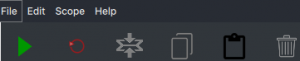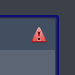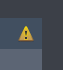# Composer BasicsThe symbols in the lower bar has the
following functionality (left to right):

• Run simulation (if the scene contains For-Loop scopes)
• Reset simulation (if the scene contains For-Loop scopes)
• Collapse/Uncollapse selected scope
• Copy
• Paste
• Clear scene

### Normalization

Wave functions can be normalized by hand or automatically by the program, although there is not a strict requirement to do so.

Discretizing the continuous normalization condition yields $\int_{-\infty}^{\infty} |\psi(x)|^2 dx = 1 \rightarrow \sum_{n=1}^N |\psi(x_n)|^2 \Delta x = 1 \Rightarrow \sum_{n=1}^N |\psi(x_n)|^2 = \frac{1}{\sqrt{\Delta x}}$.

In the discrete representation, wave functions must therefore be normalized to $\frac{1}{\sqrt{\Delta x}}$ instead of to 1.

This normalization factor is applied automatically by the program.

• Infinite(a,b) = $\begin{cases} 0, \quad a < x < b \\ \infty, \quad \text{otherwise} \end{cases}$
• Box(a,b) = $\begin{cases} 1, \quad a < x < b \\ 0, \quad \text{otherwise} \end{cases}$
• Step(a) = $\begin{cases} 0, \quad x < a \\ 1, \quad \text{otherwise} \end{cases}$
• The harmonic potential with a frequency $\omega=a$:  0.5*a^2*x^2
• Gaussian wave packet:  sqrt(sqrt(a/pi))*exp(-0.5*a*x^2)

### Expressions

Some nodes in Composer allow users to enter analytical expressions. The most common use cases are potentials and wave functions. Here are a few common expressions.A Gaussian wave packet situated in an infinite well with a slanted side. In this example the wave function is automatically normalized.

### Warning and Error node states

Error:                 Warning:Errors indicate that the node is unable to calculate its outputs, while warnings simply indicate possible oversights that may lead to surprising behavior.

### Units

Composer works with non-dimensionalized equations where effectively $\hbar = m = 1$ ('natural units')